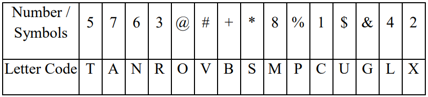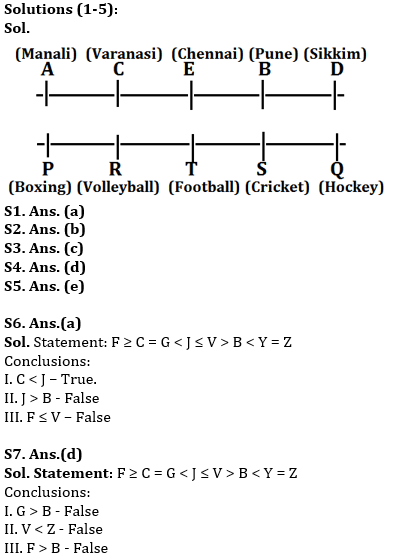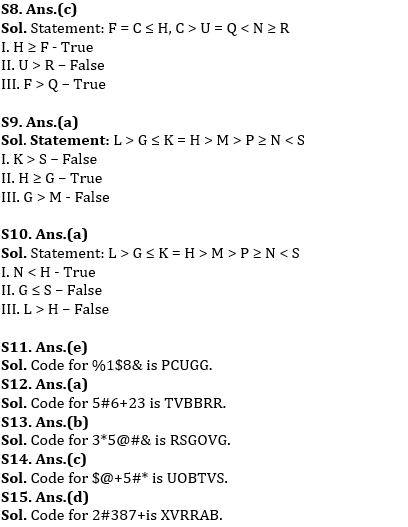Latest Banking jobs   »   ESIC Mains Syllabus 2022   »

# Reasoning Ability Quiz For RBI Assistant/ ESIC UDC Mains 2022- 8th April

Directions (1-5): Study the following information carefully and answer the question given below-
Ten persons are seating on two parallel rows. P, Q, R, S, and T are seating in row 1 and facing towards north. A, B, C, D and E are seating in row 2 and facing south. Persons who are facing north like some Sports and Persons who are facing south like some City. The one who likes Pune faces the one who sits immediate left of Q who likes Hockey. A sits at one of the extreme end of the row and likes Manali. Only two persons sit between the one who face A and the one who likes Cricket. D sits second to the left of E. P likes Boxing and faces A. The one who likes Volleyball sits third to the left of the one who faces the one who likes Sikkim. R sits second to the left of S and faces the one who likes Varanasi. C sits at one of the positions to the right of the one who likes Chennai. One of these persons likes Football. Neither E nor D face the one who likes Cricket.

Q1. Who among the following person sits immediate right of the one who likes Football ?
(a) S
(b) P
(c) R
(d) Q
(e) None of these

Q2. Who among the following sits opposite to the one who likes Cricket?
(a) A
(b) B
(c) C
(d) D
(e) None of these

Q3. Who among the following likes Varanasi?
(a) E
(b) B
(c) C
(d) A
(e) None of these

Q4. What is the position of A with respect to one who likes Pune?
(a) Third to the left
(b) Immediate right
(c) Second to the left
(d) Third to the right
(e) None of these

Q5. Which of the following statement is not true about D?
(a) D sits at the end
(b) R sits opposite to D
(c) D likes Sikkim
(d) D sits immediate right of B
(e) Both (b) and (d)

Directions (6-7): Study the data carefully and answer the questions accordingly.
‘M ) N’ means ‘M is not smaller than N’
‘M & N’ means ‘M is neither smaller than nor equal to N’
‘M ^ N’ means ‘M is neither greater than nor smaller than N’
‘M * N’ means ‘M is not greater than N’
‘M % N’ means ‘M is neither greater than nor equal to N’

Q6. Statements: G % J, B % Y ^ Z, F ) C ^ G, J * V & B
Conclusions:
I. C % J
II. J & B
III. F * V
(a) If only I follows
(b) If either I or III follows
(c) If both I and II follows
(d) If none follows
(e) If all follows

Q7. Statements: G % J, B % Y ^ Z, F ) C ^ G, J * V & B
Conclusions:
I. G & B
II. V % Z
III. F & B
(a) If only I follows
(b) If either I or III follows
(c) If both I and II follows
(d) If none follows
(e) If all follows

Directions (8-10): Study the data carefully and answer the questions accordingly.
‘X\$Y’ means ‘X is neither greater than nor smaller than Y’
‘X+Y’ means ‘X is not greater than Y’
‘X]Y’ means ‘X is not smaller than Y’
‘X&Y’ means ‘X is neither smaller than nor equal to Y’
‘X@Y’ means ‘X is neither greater than nor equal to Y’

Q8. Statements: F \$ C + H, C & U \$ Q, R + N & Q
Conclusions:
I. H ] F
II. U & R
III. F & Q
(a) If only I follows
(b) If both I and II follows
(c) If both I and III follows
(d) If none follows
(e) If all follows

Q9. Statements: K \$ H & M & P, L & G + K, P ] N @ S
Conclusions:
I. K & S
II. H ] G
III. G & M
(a) If only II follows
(b) If both I and II follows
(c) If both I and III follows
(d) If none follows
(e) If all follows

Q10. Statements: K \$ H & M & P, L & G + K, P ] N @ S
Conclusions:
I. N @ H
II. G + S
III. L & H
(a) If only I follows
(b) If both I and II follows
(c) If both I and III follows
(d) If none follows
(e) If all follows

Directions (11-15): In each question below is given a group of numbers / symbols followed by five combinations of letter codes numbered (1), (2), (3), (4) and (5). You have to find out which of the combinations correctly represents the group of numbers / symbols based on the following coding system and the conditions and mark the number of that combination as your answer:Conditions:
(1) If the third element is a prime number and the last element is a symbol then that prime number is to be used as the code for the symbol.
(2) If there are no odd numbers then the codes of the third and the fourth elements have to be interchanged.
(3) If an even number is immediately preceded by symbols then the even number is to be coded as its next number/symbol.

Q11. What is the code for %1\$8&?
(a) PCIGG
(b) PCUFG
(c) PDUGG
(d) PCMGG
(e) None of these

Q12. What is the code for 5#6+23?
(a) TVBBRR
(b) TVNBXR
(c) TVVBCR
(d) TVVNCR
(e) None of these

Q13. What is the code for 3*5@#&?
(a) R5SOVG
(b) RSGOVG
(c) RSTOVG
(d) RSTTVG
(e) None of these

Q14. What is the code for \$@+5#*?
(a) OUTBVS
(b) UBOTSV
(c) UOBTVS
(d) OUSTBV
(e) None of these

Q15. What is the code for 2#837+?
(a) VXAMMB
(b) VXMBAB
(c) XBVMBA
(d) XVRRAB
(e) None of these

Solutions#### Congratulations!Download Hindu Review of October 2021: Free PDFIncorrect details? Fill the form again here

Download Hindu Review of October 2021: Free PDF

Thank You, Your details have been submitted we will get back to you.
•Reasoning Ability Quiz For IBPS RRB PO C...
•Reasoning Ability Quiz For IBPS Clerk Pr...
•Reasoning Ability Quiz For IBPS RRB PO C...
•Reasoning Ability Quiz For IBPS RRB PO C...
•Reasoning Ability Quiz For IBPS RRB PO C...
•Reasoning Ability Quiz For IBPS Clerk Pr...